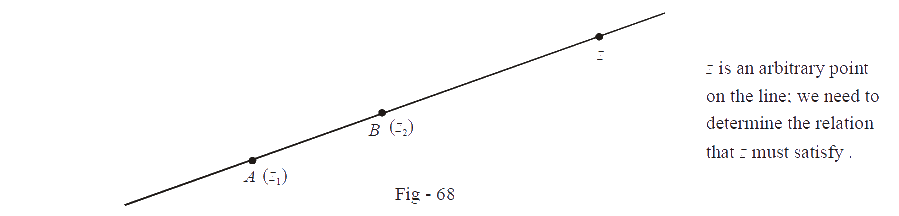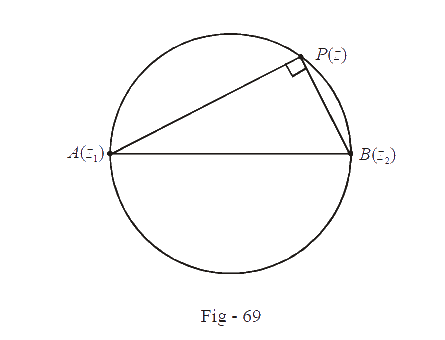# Straight Lines And Circles Using Complex Numbers

Example - 33

Find the equation of the straight line joining the points $$A(z{_1}){\text{ and }}B(z{_2}).$$

Solution: The situation is sketched in the figure below:Observe from the figure that the vectors $$z-{z_1}$$  and $$z-{z_2}$$ are either in the same direction (wherever $$z$$ lies out side the line segment $$AB$$) or they are antiparallel (whenever $$z$$ lies between $$A$$ and $$B$$). Thus, the vector $$z-{z_1}$$  can be obtained by multiplying the vector $$z-{z_2}$$  with a scalar (a real number) in all cases:

\begin{align}z - {z_1} &= s(z - {z_2}); \qquad \qquad s \in \mathbb{R}\\\Rightarrow \quad z - sz &= {z_1} - s{z_2}\\ z &= \frac{1}{{1 - s}}{z_1} - \frac{s}{{1 - s}}{z_2}\\&= t{z_1} + (1 - t){z_2}\,{\text{where }}t = \frac{1}{{1 - s}} \in \mathbb{R}\end{align}

Thus, we vary $$t$$ over all real numbers in the relation above and we’ll therefore obtain all the corresponding points on the required line.

This is the equation of the straight line in parametric form.

We can also write the required equation without involving any parameter. Since $$z - {z_2}$$  is a scalar (real) multiple of $${z_1}{\rm{ }}-{z_2}$$ ,

\begin{align}&\frac{{z - {z_2}}}{{{z_1} - {z_2}}}\,\,\,\,\,\,\,{\text{is purely}}{\text{ real}}\\\Rightarrow \qquad &\frac{{z - {z_2}}}{{{z_1} - {z_2}}} = \frac{{\bar z - {{\bar z}_2}}}{{{{\bar z}_1} - {{\bar z}_2}}}\\\Rightarrow \qquad &z({{\bar z}_1} - {{\bar z}_2}) - \bar z({z_1} - {z_2}) + {z_1}{{\bar z}_2} - {{\bar z}_1}{z_2} = 0\,\,\,\,\,\,\,\,\,\,\,\,\,\,\,\,\,\,\,\,\,...\left( 1 \right) \hfill \\\end{align}

This is the required equation in non-parametric form. How do we measure the slope of this line from the expression in (1)? Observe that the required slope is $$\tan \theta = \tan \left( {\arg ({z_1} - {z_2})} \right).$$

If we evaluate \begin{align}&\frac{{{z_1} - {z_2}}}{{\overline {{z_1} - {z_2}} }} ,\end{align} which is actually, \begin{align}\frac{{|{z_1} - {z_2}|{e^{i\theta }}}}{{|{z_1} - {z_2}|{e^{ - i\theta }}}} = {e^{2i\theta }},\end{align} we do get a measure of $$\theta$$ .  Thus, we can use \begin{align}\frac{{{z_1} - {z_2}}}{{{{\bar z}_1} - {{\bar z}_2}}}\end{align} as some form of slope. Lets call it the complex slope of the line given by (1).

Now, (1) can be simplified further. Notice that $${z_1}{\bar z_2} - {\bar z_1}{z_2}$$ can be written as $${z_1}{\bar z_2} - \overline {{z_1}{{\bar z}_2}}$$  which is equal to $$2i\,{\mathop{\rm Im}\nolimits} ({z_1}\,{\bar z_2}).$$

Therefore, (1) becomes

$$z({\bar z_1} - {\bar z_2}) - \bar z({z_1} - {z_2}) + 2i\,{\mathop{\rm Im}\nolimits}\,{z_1}{\bar z_2}) = 0$$

Multiplying both sides by $$i,$$ we get

$\begin{array}{l}z\left( {i({{\bar z}_1} - {{\bar z}_2})} \right) - \bar z\left( {i({z_1} - {z_2})} \right) - 2{\mathop{\rm Im}\nolimits} ({z_1}{{\bar z}_2}) = 0\\az + \bar a\,\bar z + b = 0 & \, & \left\{ \begin{array}{l}{\rm{where \; }}a = i({{\bar z}_1} - {{\bar z}_2})\\{\rm{so \;\;that \; }}\bar a = - i({z_1} - {z_2})\\{\rm{and\; }}b{\rm{\; is real}}\end{array} \right\}\end{array}$

The complex slope of this line \begin{align}& - \frac{{\bar a}}{a} \left( {\frac{{ - {\rm{coefficient}}\,of\,\bar z}}{{{\rm{coefficient}}\,of\,z}}\,} \right)\end{align}. This is the equation of a straight line in its most general (complex form).  From this equation try to write down the condition for the collinearity of three points in determinant form

Example - 34

(a)    If $$s{_1}\,{\rm{ and\,}}s{_2}$$ be the complex slopes of two lines, find the condition on them so that the lines are

(i) parallel      (ii)   perpendicular

(b)   Find the equation of a line perpendicular to the line $$az + \bar a\,\bar z + b = 0,$$ passing through $${z_1}$$ .

Solution: (a) Let the actual slopes be $$\tan {\theta _1}$$ and $$\tan {\theta _2}$$

The complex slopes are $${s_1} = {e^{2i{\theta _1}}}$$ and $${s_2} = {e^{2i{\theta _2}}}$$

(i)  For parallel lines, $${\theta _1} = {\theta _2}$$ . Therefore,

${s_1} = {s_2}$

(ii)  For perpendicular lines, \begin{align}{\theta _1} - {\theta _2} = \frac{\pi }{2}\end{align}

\begin{align}& \Rightarrow \qquad 2({\theta _1} - {\theta _2}) = \pi \\\\ &\Rightarrow \qquad {e^{i2({\theta _1} - {\theta _2})}} = {e^{i\pi }} = - 1\\\\ &\Rightarrow \qquad {s_1} \cdot s_2^{ - 1} = - 1\\\\ &\Rightarrow \qquad {s_1} + {s_2} = 0 \end{align}

(b) The complex slope of the original line is

${s_1} = - \frac{{\bar a}}{a}$

The complex slope of the perpendicular line will be

${s_2} = \frac{{z - {z_1}}}{{\bar z - {{\bar z}_1}}}$

Since, the lines are perpendicular, $${s_1} + {s_2} = 0$$

\begin{align} &\Rightarrow \qquad \frac{{z - {z_1}}}{{\bar z - {{\bar z}_1}}} - \frac{{\bar a}}{a} = 0\\ &\Rightarrow\qquad a(z - {z_1}) - \bar a(\bar z - {{\bar z}_1}) = 0\\& \Rightarrow\qquad az - \bar a\bar z - (a{z_1} - \bar a{{\bar z}_1}) = 0\\ &\Rightarrow \qquad az - \bar a\,\bar z - 2i\,{\mathop{\rm Im}\nolimits} (a{z_1}) = 0\end{align}

Example - 35

Let $$A({z_1})$$ and $$B({z_2})$$ be arbitrary points in the complex plane. Find the equation of the circle having $$AB$$ as a diameter.

Solution: Let $$P(z)$$ be an arbitrary point lying on the required circle as shown in the figure belowWe can now take two approaches:

(i) We know that the angle in a semi-circle is a right angle. Therefore,

$\begin{array}{l} A{P^2} + P{B^2} = A{B^2}\\ |z - {z_1}{|^2} + |z - {z_2}{|^2} = \,|{z_1} - {z_2}{|^2} \end{array}$

This is a possible equation of the required circle. All points lying on the circle will satisfy this equation

(ii)  Applying rotation $$(\overrightarrow {PA} \buildrel {} \over \longrightarrow \overrightarrow {PB} ),$$ we get

\begin{align}&\qquad\quad\;\; \frac{{{z_2} - z}}{{{z_1} - z}} = \frac{{|{z_2} - z|}}{{|{z_1} - z|}}{e^{i\pi /2}} = ik,\;\;\;\;\;\; k \in \mathbb{R}\\ &\Rightarrow \qquad \frac{{{z_2} - z}}{{{z_1} - z}}{\rm{\;is\; purely\; imaginary}}\\&\Rightarrow \qquad \frac{{z - {z_2}}}{{z - {z_1}}} + \frac{{\bar z - {{\bar z}_2}}}{{\bar z - {{\bar z}_1}}} = 0\\&\Rightarrow \qquad (z - {z_1})(\bar z - {\bar z_2}) + (\bar z - {\bar z_1})(z - {z_2}) = 0\end{align}

This is another possible equation of the required circle.

Example - 36

Find the general equation of a circle in complex form

Solution: Let us consider an arbitrary circle with centre  $$z{_0}$$ and radius $$r.$$

\begin{align}&\qquad \;\;|z - {z_0}| = r\\&\Rightarrow \quad |z - {z_0}{|^2} = {r^2}\\&\Rightarrow \quad (z - {z_0})(\bar z - {{\bar z}_0}) = {r^2}\\&\Rightarrow \quad z\bar z - z{{\bar z}_0} - \bar z{z_0} + {z_0}{{\bar z}_0} - {r^2} = 0\\ &\Rightarrow \quad|z{|^2} + a\,z + \bar a\,\bar z + k = 0 \qquad \qquad \text{where}\,a = - {{\bar z}_0}\;\text{and}\;k = |{z_0}{|^2} - {r^2} \end{align}

This is the general equation that we required. Given this form, we can easily deduce that the centre will be $$- \bar a$$ and the radius will be $$\sqrt {|{z_0}{|^2} - k}$$ .

Learn from the best math teachers and top your exams

• Live one on one classroom and doubt clearing
• Practice worksheets in and after class for conceptual clarity
• Personalized curriculum to keep up with school